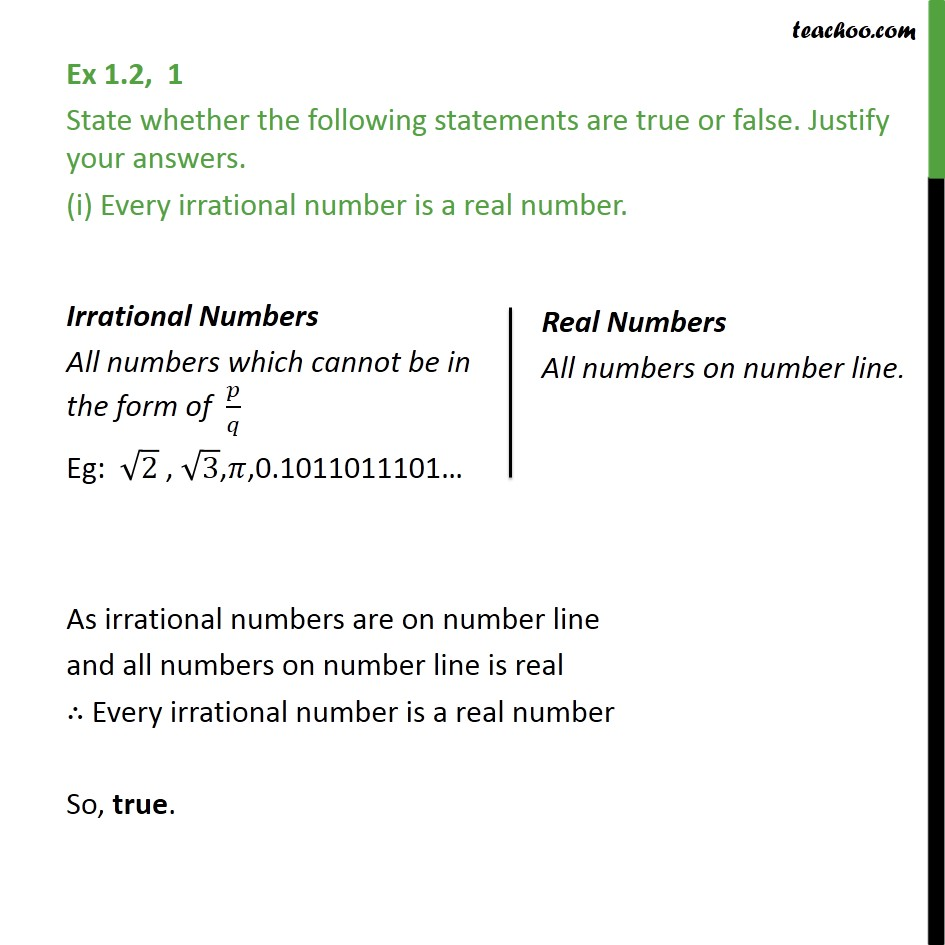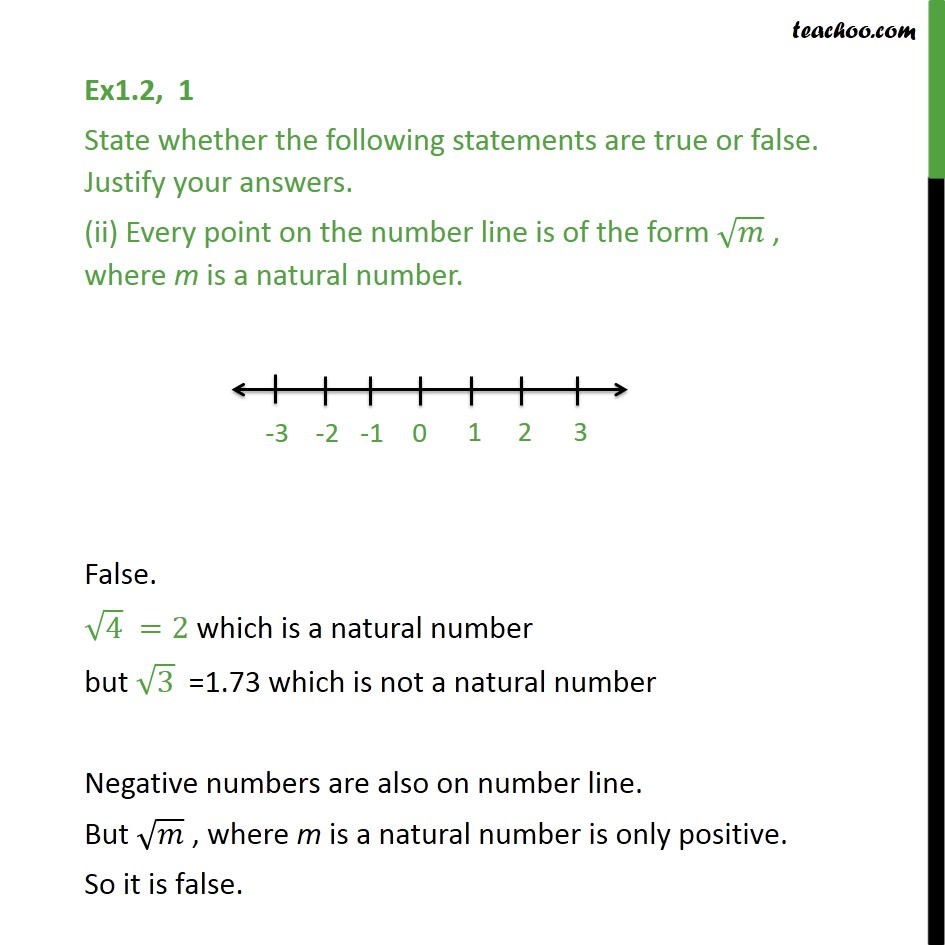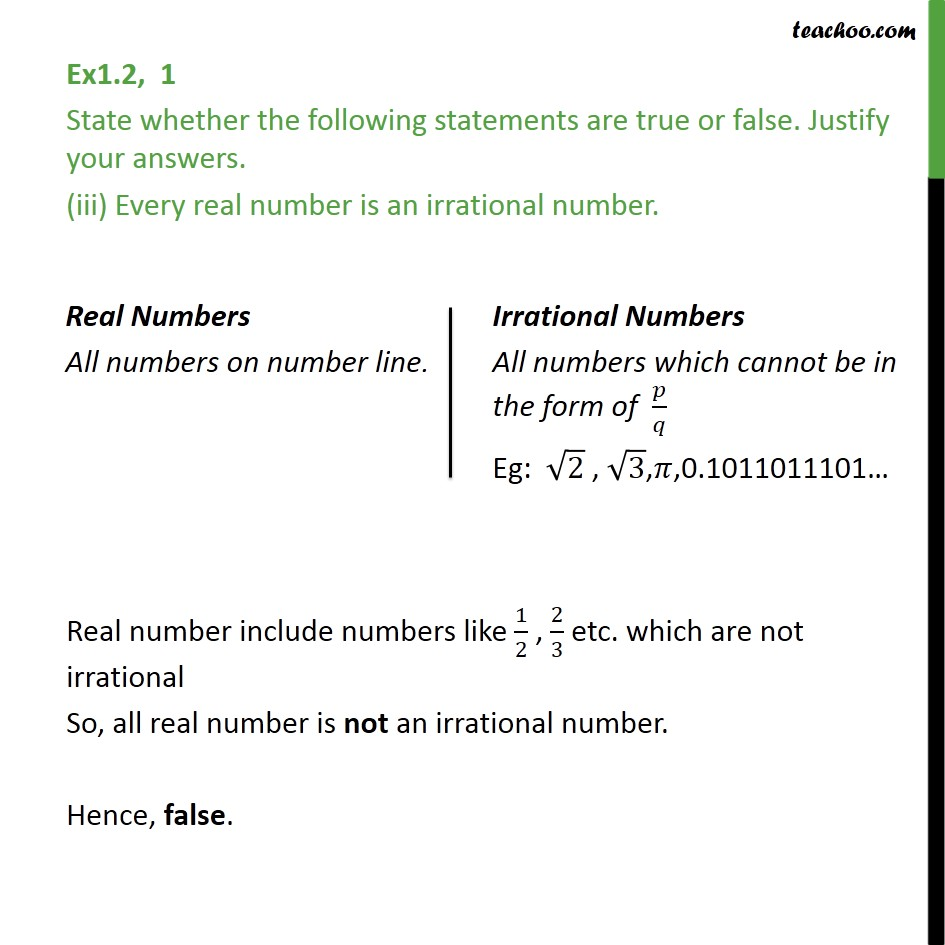Learn All Concepts of Chapter 1 Class 9 - FREE. Check - Chapter 1 Class 9 Maths1. Chapter 1 Class 9 Number Systems
2. Serial order wise
3. Ex 1.2

Transcript

Ex 1.2, 1 State whether the following statements are true or false. Justify your answers. (i) Every irrational number is a real number. As irrational numbers are on number line and all numbers on number line is real ∴ Every irrational number is a real number So, true. Ex1.2, 1 State whether the following statements are true or false. Justify your answers. (ii) Every point on the number line is of the form √𝑚 , where m is a natural number. False. √4 =2 which is a natural number but √3 =1.73 which is not a natural number Negative numbers are also on number line. But √𝑚 , where m is a natural number is only positive. So it is false. Ex1.2, 1 State whether the following statements are true or false. Justify your answers. (iii) Every real number is an irrational number. Real number include numbers like 1/2 , 2/3 etc. which are not irrational So, all real number is not an irrational number. Hence, false.

Ex 1.2